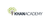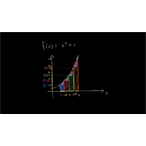# Class 11 Physics (India): Riemann approximation introduction

Type product
Tijdsduur

## Class 11 Physics (India): Riemann approximation introductionKhan Academy

Tip: laat jezelf inspireren, bekijk hier de video!### Beschrijving

Approximating the area under a curve using some rectangles.  This is called a "Riemann sum".

## Class 11 Physics (India)

Let's learn, practice, and master topics of class 11 physics (NCERT) starting with kinematics and then moving to dynamics with Newton's laws of motion, work, energy, and power. Let's then use these as the foundation to learn about centre of mass, rotational motion, gravitation, solids, fluids, thermodynamics, and oscillations and waves.

## Riemann sums

Riemann sums is the name of a family of methods we can use to approximate the area under a curve. Learn about the different ways and how they are constructed.

## Topic: Science

Learn about all the sciences, from physics, chemistry an…

Lees de volledige beschrijving

### Veelgestelde vragen

Er zijn nog geen veelgestelde vragen over dit product. Als je een vraag hebt, neem dan contact op met onze klantenservice.

Approximating the area under a curve using some rectangles.  This is called a "Riemann sum".

## Class 11 Physics (India)

Let's learn, practice, and master topics of class 11 physics (NCERT) starting with kinematics and then moving to dynamics with Newton's laws of motion, work, energy, and power. Let's then use these as the foundation to learn about centre of mass, rotational motion, gravitation, solids, fluids, thermodynamics, and oscillations and waves.

## Riemann sums

Riemann sums is the name of a family of methods we can use to approximate the area under a curve. Learn about the different ways and how they are constructed.

## Topic: Science

Learn about all the sciences, from physics, chemistry and biology, to cosmology and astronomy, across hundreds of videos, articles and practice questions.

Er zijn nog geen ervaringen.

### Deel je ervaring

Heb je ervaring met deze cursus? Deel je ervaring en help anderen kiezen. Als dank voor de moeite doneert Springest € 1,- aan Stichting Edukans.

Er zijn nog geen veelgestelde vragen over dit product. Als je een vraag hebt, neem dan contact op met onze klantenservice.